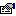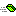## BigInteger

Represents an unlimited size signed integer value.

### Public:

#### Properties:

NameDescriptionIsEven (get) Returns if the value is an even number.IsOne (get) Returns if the value is equal to one.IsZero (get) Returns if the value is equal to zero.Precision (get) Returns the number of bytes being used to represent the number.Sign (get) Returns the sign of the number this instance represents.

#### Methods:

NameDescriptionAbsolute Returns the absolute (positive or zero) value of this instance.Add Adds a value to this instance of BigInteger.BitwiseAnd Peforms a bitwise AND of this BigInteger instance and the value passed in.BitwiseAndNot Peforms a bitwise AND of this BigInteger instance and the NOT value passed in.BitwiseNot Peforms a bitwise NOT of this BigInteger instance and the value passed in.BitwiseOr Performs a bitwise OR of this BigInteger instance and the value passed in.BitwiseXor Performs a bitwise XOR of this BigInteger instance and the value passed in.ClearBit Clears the specified bit of the BigInteger instance to 0.CompareTo Compares two BigInteger instance to each other returning their relation.Divide Divides this BigInteger instance by the supplied divisor.DivRem Divides this value by the value, returning the quotient and remainding.Equals Determines if this BigInteger instance is equal to the other value.Factorial Returns the factorial of this BigInteger value.FlipBit Flips the specified bit, toggling between True and False.GetHashCode Returns a pseudo-unique number identifying this instance.GreatestCommonDivisor Returns the greatest common divisor between this instance and the passed in value.Max Returns the maximum of this instance and a second BigInteger instance.Min Returns the mininum of this instance and a second BigInteger instance.Modulus Performs a modulus operation, returning the remainder.Multiply Multiplies this instance of BigInteger by the supplied multiplier.Negate Returns the opposite signed value of this BigInteger instance.Pow Raises the BigInteger value to the specified power.SetBit Sets the specified bit of the BigInteger instance to 1.ShiftLeft Performs a left shift operation on the BigInteger instance.ShiftRight Performs a bit shifting operation to the the right.SquareRoot Returns the integer square root of a BigInteger.Subtract Subtracts a value to this instance of BigInteger.TestBit Tests a specific bit in the number to determine if it is set (1) or not (0).ToByteArray Returns a byte array representing the number.ToString Returns a human readable string of characters representing the number.

#### Remarks

The BigInteger can represent a positive or negative value limited in size only by memory.

Negative values are represented in a twos-complement fashion mimicking the way the hardware and VB uses signed integer values.

The value is represented internally as an array of Integers. This was selected over Bytes because of the speed increase with working with multiple bytes simultaneously. However, Integers are never exposed outside of the class, only byte arrays.